# ISEE Lower Level Math : How to find the area of a rectangle

## Example Questions

1 2 3 4 5 7 Next →

### Example Question #74 : Plane Geometry

Find the area of a rectangle with a length of 16in and a width that is half the length.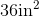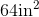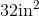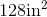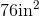Explanation:

To find the area of a rectangle, we will use the following formula: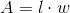where l is the length and w is the width of the rectangle.

Now, we know the length of the rectangle is 16in.  We also know the width is half of the length.  Therefore, the width is 8in.

Knowing this, we can substitute into the formula.  We get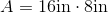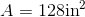### Example Question #75 : Plane Geometry

Find the area of the following rectangle: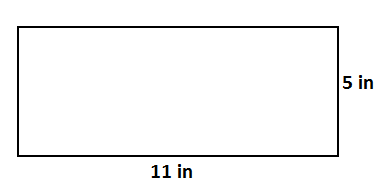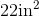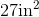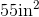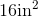Explanation:

To find the area of a rectangle, we will use the following formula:where l is the length and w is the width of the rectangle.

Now, given the rectanglewe can see the length is 11in and the width is 5in.  Knowing this, we can substitute into the formula.  We get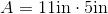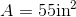### Example Question #76 : Plane Geometry

Find the area of a rectangle with a width of 4in and a length that is two times the width.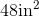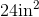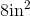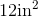Explanation:

To find the area of a rectangle, we will use the following formula:where l is the length and w is the width of the rectangle.

Now, we know the width of the rectangle is 4in.  We also know the length of the rectangle is two times the width.  Therefore, the length is 8in.  So, we get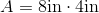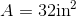1 2 3 4 5 7 Next →